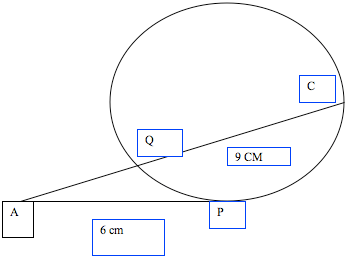SEARCH HOMEMath Central Quandaries & QueriesAP is a tangent at P to a circle centre O, where AP=6cm. The straight line AQC is such that QC= 9cm. Find the length, in cm of AQ.Fawad's problem came with this diagram.There is an important theorem about circles that everybody should know:
If A is any point on the outside of a circle, let AP be one of its tangents (with P the point of tangency), and let any secant AQ meet the circle again at C. Then the lengths satisfy
AP2 = AQ X AC.

ChrisMath Central is supported by the University of Regina and The Pacific Institute for the Mathematical Sciences.